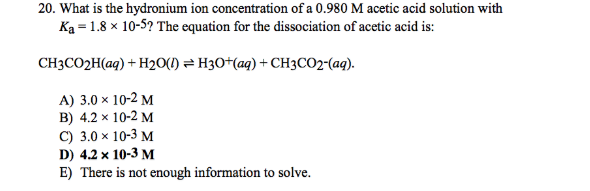# Problem: What is the hydronium ion concentration of a 0.980 M acetic acid solution with Ka = 1.8 x 10-5? The equation for the dissociation of acetic acid is: CH3CO2H (aq) + H2O (l) ⇌ H3O+ (aq) + CH3CO2- (aq). a. 3.0 times 10-2 M b. 4.2 times 10-2 M c. 3.0 times 10-3 M d. 42 times 10-3 M e. There is not enough information to solve.

###### FREE Expert Solution
87% (366 ratings)###### Problem Details

What is the hydronium ion concentration of a 0.980 M acetic acid solution with Ka = 1.8 x 10-5? The equation for the dissociation of acetic acid is:

CH3CO2H (aq) + H2O (l) ⇌ H3O+ (aq) + CH3CO2- (aq).

a. 3.0 times 10-2

b. 4.2 times 10-2

c. 3.0 times 10-3

d. 42 times 10-3

e. There is not enough information to solve.